## RD Sharma Class 9 Solutions Chapter 4 Algebraic Identities Ex 4.2

These Solutions are part of RD Sharma Class 9 Solutions. Here we have given RD Sharma Class 9 Solutions Chapter 4 Algebraic Identities Ex 4.2

Question 1.
Write the following in the expanded form: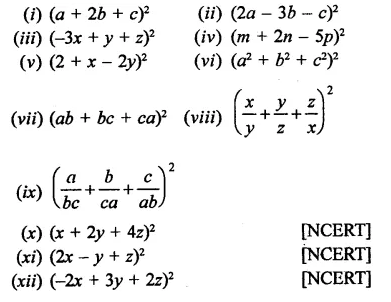Solution: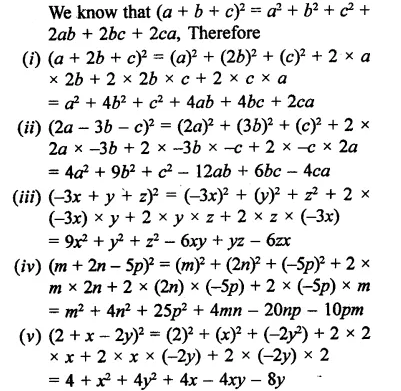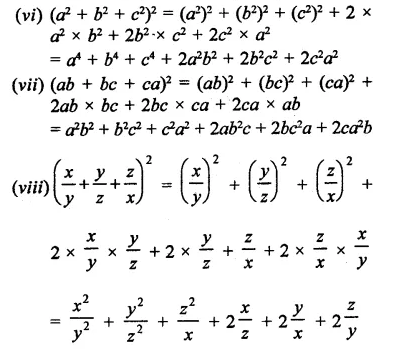Question 2.
If a + b + c = 0 and a2 + b2 + c2 = 16, find the value of ab + be + ca.
Solution:
a + b+ c = 0
Squaring both sides,
(a + b + c)2 = 0
⇒ a2 + b2 + c2 + 2(ab + bc + ca) = 0
16 + 2(ab + bc + c) = 0
⇒ 2(ab + bc + ca) = -16
⇒  ab + bc + ca =-$$\frac { 16 }{ 2 }$$ = -8
∴ ab + bc + ca = -8

Question 3.
If a2 + b2 + c2 = 16 and ab + bc + ca = 10, find the value of a + b + c.
Solution:
(a + b + c)2 = a2 + b2 + c2 + 2(ab + bc + ca)
= 16 + 2 x 10
= 16 + 20 = 36
= (±6)2
∴ a + b + c = ±6

Question 4.
If a + b + c = 9 and ab + bc + ca = 23, find the value of a2 + b2 + c2.
Solution:
(a + b + c)2 = a2 + b2 + c2 + 2(ab + bc + ca)
⇒ (9)2 = a2 + b2 + c2 + 2 x 23
⇒ 81= a2 + b2 + c2 + 46
⇒  a2 + b2 + c2 = 81 – 46 = 35
∴
a2 + b2 + c2 = 35

Question 5.
Find the value of 4x2 + y2 + 25z2 + 4xy – 10yz – 20zx when x = 4, y = 3 and z = 2.
Solution:
x = 4, y – 3, z = 2
4x2 + y2 + 25z2 + 4xy – 10yz – 20zx
= (2x)2 + (y)2 + (5z)2 + 2 x2 x x y-2 x y x 5z – 2 x 5z x 2x
= (2x + y- 5z)2
= (2 x 4 + 3- 5 x 2)2
= (8 + 3- 10)2
= (11 – 10)2
= (1)2 = 1

Question 6.
Simplify:
(i)  (a + b + c)2 + (a – b + c)2
(ii) (a + b + c)2 –  (a – b + c)2
(iii) (a + b + c)2 +   (a – b + c)2 + (a + b – c)2
(iv) (2x + p – c)2 – (2x – p + c)2
(v) (x2 + y2 – z2)2 – (x2 – y2 + z2)2
Solution: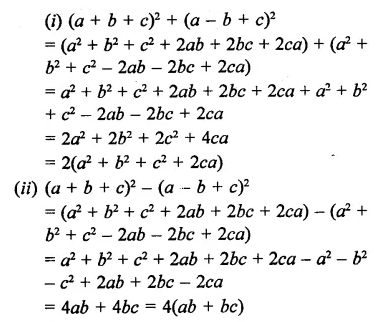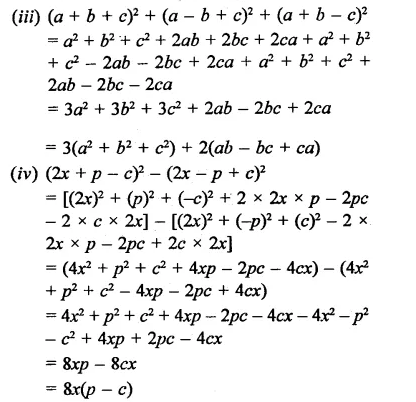Question 7.
Simplify each of the following expressions:Solution: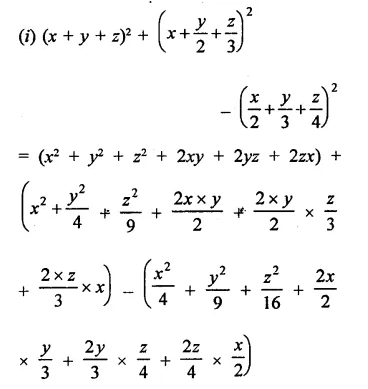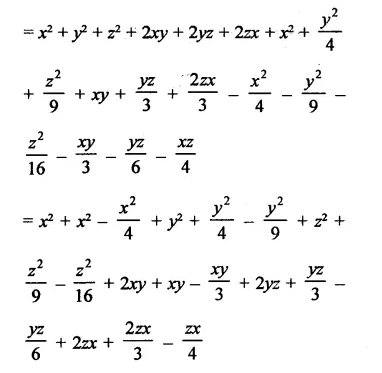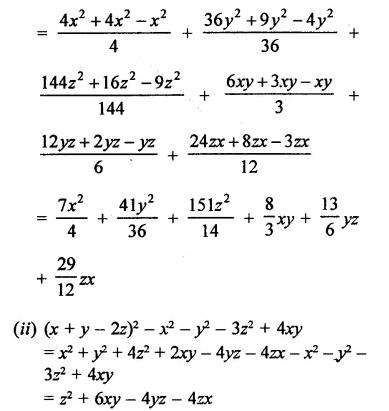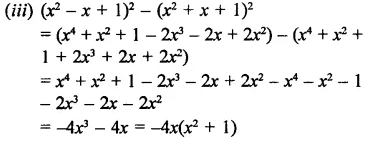Hope given RD Sharma Class 9 Solutions Chapter 4 Algebraic Identities Ex 4.2 are helpful to complete your math homework.

If you have any doubts, please comment below. Learn Insta try to provide online math tutoring for you.